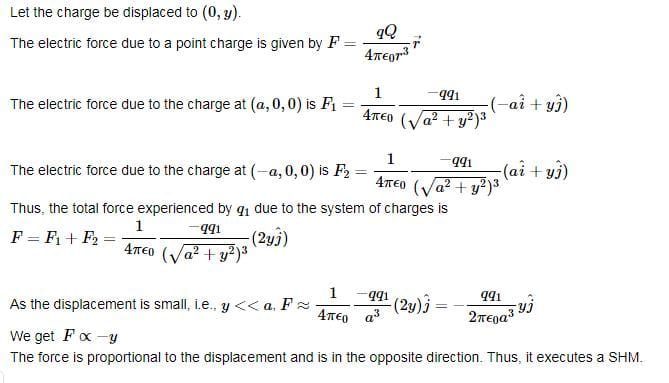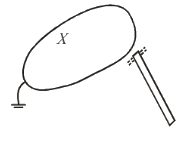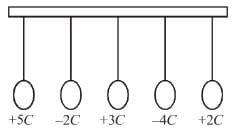# Electric Charge MCQ Level - 2

## 10 Questions MCQ Test Electricity & Magnetism | Electric Charge MCQ Level - 2

Description
Attempt Electric Charge MCQ Level - 2 | 10 questions in 45 minutes | Mock test for IIT JAM preparation | Free important questions MCQ to study Electricity & Magnetism for IIT JAM Exam | Download free PDF with solutions
QUESTION: 1

### Effective mass of an electron in an semiconductor

Solution:

The correct answer is: can never be negative

QUESTION: 2

Solution:
QUESTION: 3

### A charge +q is placed (a, 0, 0) and another +q is placed (-a, 0, 0). A charge –q is placed at the origin. If it is slightly displaced along y-axis. Then

Solution:The correct answer is: It will execute SHM

QUESTION: 4

The SI unit of electric flux is

Solution:

Electric flux has SI units of volt metres (V m), or, equivalently, newton metres squared per coulomb (N m2 C−1). Thus, the SI base units of electric flux are kg·m3·s−3·A−1

QUESTION: 5

Which of the following statement concerning lightning is/are correct?
1. It is a result of charging
2. The charge at the bottom of the clouds and those induced on the top of the roofs of buildings form a very strong electric field.
3. Lightning cause the thunder we hear

Solution:

a) Lightning is a naturally occurring electrostatic discharge during which two electrically charged regions in the atmosphere or ground temporarily equalize themselves, causing the instantaneous release of as much as one gigajoule of energy.

b) The charge at the bottom of the clouds and those induced on the top of the roofs of buildings form a very strong electric field.

c) Lightning causes thunder, a sound from the shock wave which develops as gases in the vicinity of the discharge experience a sudden increase in pressure.

QUESTION: 6

A negative charged rod is held near a conductor X that is earthed shown in the figure. Here X isSolution:

The correct answer is: Positively charged and at earth potential

QUESTION: 7

Which of the following should not be done during lightning.
1. Stay in a building with a lightning conductor installed on the roof.
2. Stand near a window with a metal frame.
3. Crouch down in an open field.

Solution:

1 and 3 are the preventive measures to stay safe from lightning

QUESTION: 8

The diagram shows five charged metal spheres suspended by string. The charge of each sphere is +5C, –2C, +3C, –4C and +2C as shown. If the five spheres simultaneously contact together. Find the net charge on them.Solution:

For finding the net charge we will add all the charges on all spheres.

= +5C + (-2C) + (+3C) + (-4C) + (2C)

QUESTION: 9

Two spheres P and Q are supported on two insulated stands and placed next to each other. Sphere P has a charge of +10 and sphere Q is neutral which of the following statement is correct?

Solution:

The correct answer is: After some time, Q is still neutral

QUESTION: 10

A charged of Q coulomb is placed on a solid piece of metal of irregular shape the charge will distribute itself.

Solution:

The correct answer is: Such that the potential energy of the system is minimisedUse Code STAYHOME200 and get INR 200 additional OFF Use Coupon Code